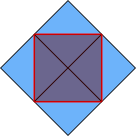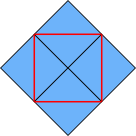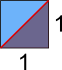#### You may also like### Hallway Borders

What are the possible dimensions of a rectangular hallway if the number of tiles around the perimeter is exactly half the total number of tiles?### Square Pegs

Which is a better fit, a square peg in a round hole or a round peg in a square hole?### Boxed In

A box has faces with areas 3, 12 and 25 square centimetres. What is the volume of the box?

# Diagonal Side

##### Age 11 to 14 ShortChallenge Level
Measuring using unit squaresThe side length of the square is equal to the diagonal of the unit square, as shown in the diagram on the right, where the red length is the side of the new square and the blue square is a unit square.Sticking four of these together, as shown on the left, draws the whole square. The unit squares fit perfectly together as the red lines split them in half, and so each angle is 90$^\text o$ or 45$^\text o$.

The area of the square is shaded in the diagram on the right. It contains four half-unit-squares - so its area is four half-units, or two whole units.

Using Pythagoras' Theorem to find the side lengthIf this square is a unit square, then its diagonal, shown in red, will be the side length of the square.

Applying Pythagoras' Theorem to the triangle will give us information about this length, here called $c$: $1^2+1^2=c^2\Rightarrow2=c^2$

But $c^2$ is the area of the square with side length $c$. So if $c^2=2$, then the area is $2$.

You can find more short problems, arranged by curriculum topic, in our short problems collection.